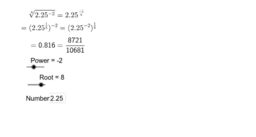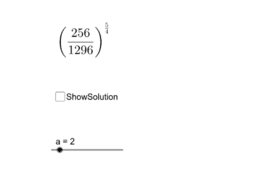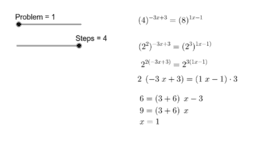# Exponents

Author:
nnhsmath
Topic:
Exponent
Some practice for Math 501 exponents. The first worksheet gives you practice with positive and negative fractional exponents. The second presents some problems related to the first worksheet. And the third worksheet consists of problems with algebraic expressions as exponents and you have to solve for x. To get a new set of problems, just reload the worksheet and get a new set of 10 problems
•### Fractional Exponents

•### Fractions and Exponents: Problems

•